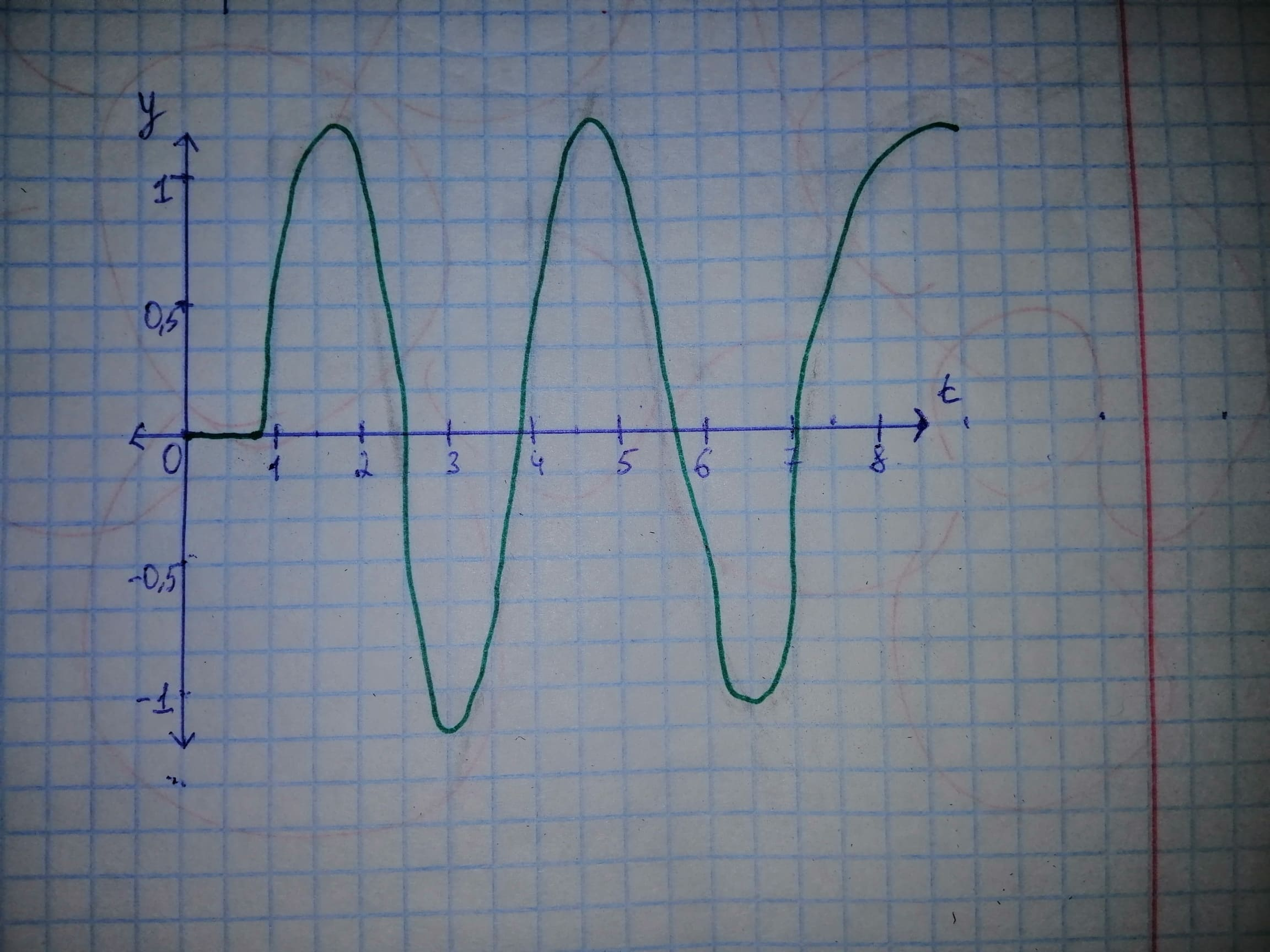# The solution of the initial value problem y'' + 4y=2delta(t - pi/4), y(0)=0, y'(0)=0 and draw the graph of the solution.Josalynn 2020-11-23 Answered
The solution of the initial value problem and draw the graph of the solution.
You can still ask an expert for help

• Questions are typically answered in as fast as 30 minutes

Solve your problem for the price of one coffee

• Math expert for every subject
• Pay only if we can solve itDelorenzoz

Let the differential equation
Applying the Laplace transform to the differential equation,

By using and

Using the initial conditios

Now, applying the inverse Laplace transformation on both sides,

By using and properties of inverse Laplace,

Therefore, the solution of the initial value problem
is

By using
For
For
Thuse, the graph of the solution is as below: